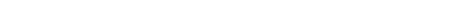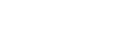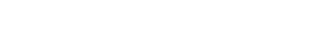AP Calculus Review: Sum and Difference Rules

The sum and difference rules tell us how to take derivatives of functions that have more than a single term. They are some of the most frequently-used rules, so much so that we tend to use them automatically without even realizing it.

The Sum and Difference Rules

Simply put, the derivative of a sum (or difference) is equal to the sum (or difference) of the derivatives.

More precisely, suppose f and g are functions that are differentiable in a particular interval (a, b). Then the sum f + g and the difference fg are both differentiable in that interval, andExample: Unknown Profit Function

Let C(x) be the cost of producing x items, and R(x) be the revenue generated from selling x items. Then the profit generated from producing and selling x items is P(x) = R(x) – C(x). Suppose we know the following data.

C(1000)C '(1000)R(1000)R '(1000)
\$7800\$8.50\$10,320\$7.80

Find the value of P ‘(1000) and interpret its meaning. Should the company produce more or fewer than 1000 items based on your analysis?

Use the difference rule:Because the derivative measures rate of change, we conclude that the company would lose 70 cents per each additional item produced and sold beyond 1000. Since increasing the production level results in less profit, the company should probably scale back production to some amount fewer than 1000 items.

Example: Polynomials

We rarely encounter the sum and difference rules in isolation. Together with the power rule and constant multiple rule, these rules allow you to differentiate any polynomial.

Find f ‘(x) if f(x) = 7x5 + 3x2 – 4x + 12.Therefore, f ‘(x) = 35x4 + 6x – 4.

Note, in practice you do not have to write out each step like this every time. Once you become familiar with the rules, you can get to the answer in one step — at least for simple functions like polynomials!

Example: General Functions

The rules work the same way even in more complicated situations.

FindIn this case, it helps to first rewrite the original function so that it is easier to take the derivative.Now we have a sum of two terms! Use the sum rule to find the derivative.Summary

The sum and difference rules are just two of the many differentiation rules that you’ll need to master in order to succeed on the AP Calculus exams. Check out this Calculus Review: Dervative Rules for a more complete picture.

Improve your SAT or ACT score, guaranteed. Start your 1 Week Free Trial of Magoosh SAT Prep or your 1 Week Free Trial of Magoosh ACT Prep today!Author

•Shaun earned his Ph. D. in mathematics from The Ohio State University in 2008 (Go Bucks!!). He received his BA in Mathematics with a minor in computer science from Oberlin College in 2002. In addition, Shaun earned a B. Mus. from the Oberlin Conservatory in the same year, with a major in music composition. Shaun still loves music -- almost as much as math! -- and he (thinks he) can play piano, guitar, and bass. Shaun has taught and tutored students in mathematics for about a decade, and hopes his experience can help you to succeed!